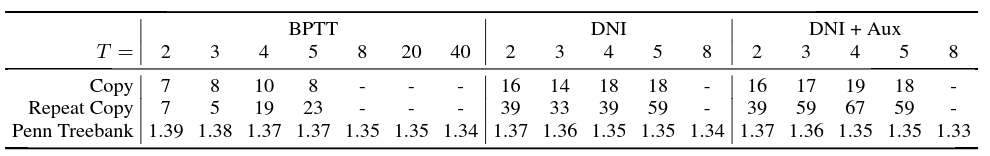# 论文笔记：Decoupled Neural Interfaces using Synthetic Gradients

• Forward Locking: 前一层没有用输入完成计算，后一层无法进行根据输入进行计算
• Update Locking: 如果一个网络层依赖的层没有完成前馈计算，该层无法进行更新
• Backward Locking: 如果一个网络层依赖的层没有完成反向传播，该层无法进行更新

• 由多个异步模块组成的复杂的系统(这个不懂……)
• 分布式模型，模型的一部分共享给多个下游客户端使用，最慢的客户端将会成为模型更新速度的短板

$\begin{array}{rcl} \theta_{i} &\leftarrow& \theta_{i} - \alpha\delta_{i}\frac{\partial h_{i}}{\partial \theta_{i}} \\ &\leftarrow& \theta_{i} - \alpha\delta_{N}\frac{\partial h_{i}}{\partial \theta_{i}}\cdot \prod_{j=0}^{N-i-1}\frac{\partial h_{N-j}}{\partial h_{N-j-1}}\end{array}$

$\begin{array}{rcl} \delta_{i} &\sim& \hat{\delta_{i}} \\ &=& M_{i+1}(h_{i})\end{array}$

$\delta_{i} = \hat{\delta}_{i+1}\frac{\partial h_{i+1}}{\partial h_{i}}$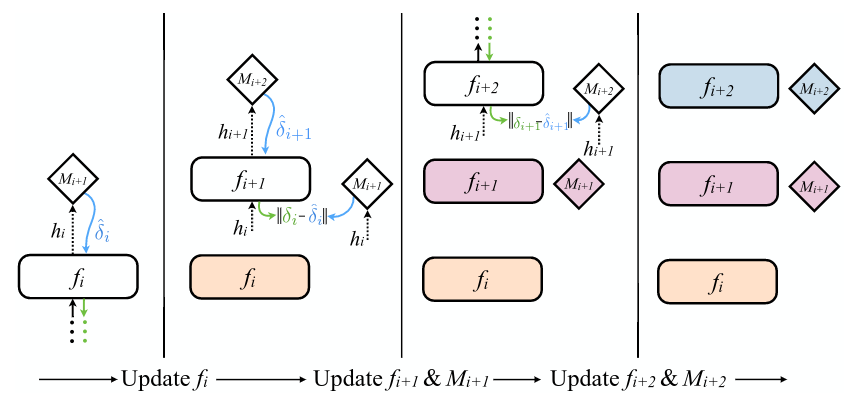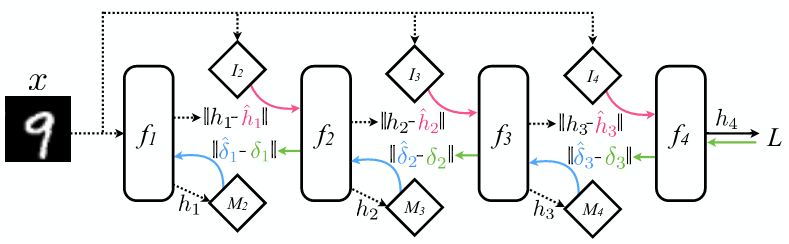DNI 的思想除了用在前馈神经网络上，也可以用于循环神经网络(Recurrent Neural Network, RNN)的训练上面，因为 RNN 在时间维度上展开后，其实就相当于是一个前馈神经网络了。而且由于应用 DNI 的模型，最多只有两层的网络层依赖，那么在用于 RNN 训练时，可以不用将 RNN 完全展开，而是可以以两个 time step 为最小单元进行展开，即一次只展开两个 time step，这样在存储上的消耗也可以被降低。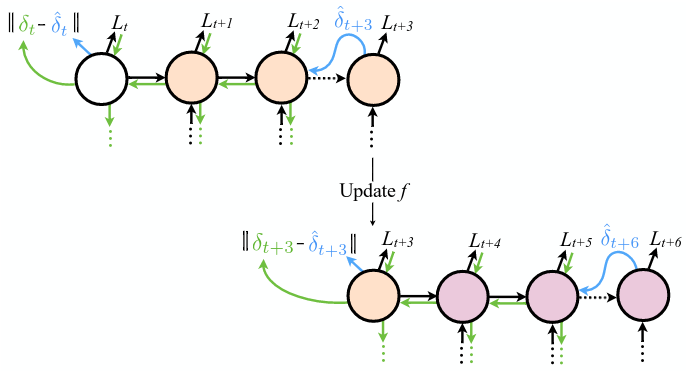Although we have explicitly described the application of DNI for communication between layers in feedforward networks, and between recurrent cores in recurrent networks, thereis nothing to restrict the use of DNI for arbitrary network graphs. The same procedure can be applied toany network or collection of networks, any number of times.

• 那个额外的模型 M 的具体细节？
• 增加了额外的模型 M ，相当于增加了参数数量，是不是更容易过拟合了？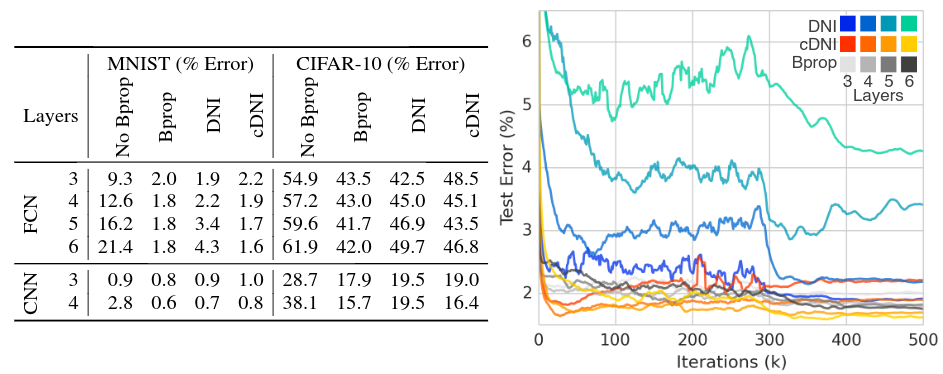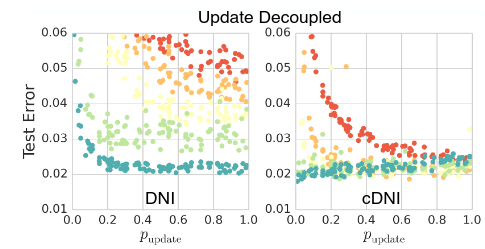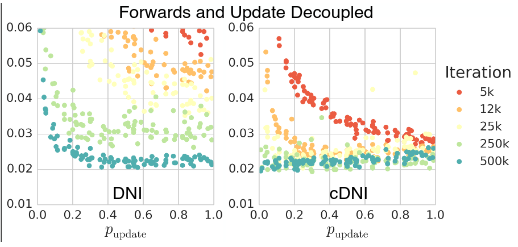• Copy: 读入 N 个字符，然后将这 N 个字符原样输出，有点类似 char-level language model 和 autoencoder。
• Repeat Copy: 读入 N 个字符，以及一个表示重复次数的数字 R，然后重复输出 R 次这 N 个字符构成的序列。
• char-level language modeling: (持续地)读取一个字符，并预测下一个字符。